# Long scenario & requirements

``````%matplotlib widget

import pickle
import numpy as np
from pacti.contracts import PolyhedralIoContract
from matplotlib.figure import Figure
from matplotlib.backends.backend_pdf import PdfPages
from typing import Tuple
from contract_utils import check_tuple, Schedule
from plot_utils import plot_steps
``````

This notebook demonstrates the visualization of a few schedulable combinations of the 20-step scenarios variants for the operational requirement variants.

``````f20 = open('results20.data', 'rb')
f20.close()

print(f"Failure results: {len(results20)}")
print(f"Successful results: {len(results20)}")
``````
``````Failure results: 3970
Successful results: 30
``````

We focus on showing the range of possible values for the battery state-of-charge at each step in the scenario. We also show the uncertainties associated with each step function across all 3 viewpoints: power, science and communication, and navigation. The operational requirements specify constraints on initial conditions and lower bounds at each step. Finally, we also compute the average batter state-of-charge as a figure of merit computed as a min/max optimization.

``````def showRange(name: str, range: Tuple[float, float]) -> str:
return f"{name}=[{range:.3g},{range:.3g}]\n"

def plot_results20(index:int, var:str) -> Figure:
schedule: Schedule = results20[index]
ranges = schedule.scenario
dsn_cons = ranges
chrg_gen = ranges
sbo_cons = ranges
tcmh_cons = ranges
tcmdv_cons = ranges
dsn_speed = ranges
sbo_gen = ranges
dsn_noise = ranges
chrg_noise = ranges
sbo_imp = ranges
tcm_dv_noise = ranges
tcm_dv_progress = ranges

op_reqs: np.ndarray = schedule.reqs
c: PolyhedralIoContract = schedule.contract
fsoc = " + ".join([f"0.05 output_soc{i}" for i in range(1, 21)])
max_soc = c.optimize(fsoc, maximize=True)
if max_soc is None:
max_soc = -1

min_soc = c.optimize(fsoc, maximize=False)
if min_soc is None:
min_soc = -1

u_bounds = check_tuple(c.get_variable_bounds("output_u20"))
r_bounds = check_tuple(c.get_variable_bounds("output_r20"))
c_bounds = check_tuple(c.get_variable_bounds("output_c20"))

text = \
"* Power uncertainties\n" + \
showRange("        chrg_gen", chrg_gen) + \
showRange("        dsn_cons", dsn_cons) + \
showRange("        sbo_cons", sbo_cons) + \
showRange("       tcmh_cons", tcmh_cons) + \
showRange("      tcmdv_cons", tcmdv_cons) + \
"* Science uncertainties\n" + \
showRange("         sbo_gen", sbo_gen) + \
showRange("       dsn_speed", dsn_speed) + \
showRange("         sbo_imp", sbo_imp) + \
showRange("       dsn_noise", dsn_noise) + \
showRange("      chrg_noise", chrg_noise) + \
showRange("    tcm_dv_noise", tcm_dv_noise) + \
showRange(" tcm_dv_progress", tcm_dv_progress) + \
"* Initial conditions\n" + \
f"     battery soc={op_reqs:.3g}\n" + \
f"    science data={op_reqs:.3g}\n" + \
f"   traj. est. u.={op_reqs:.3g}\n" + \
"* Constraints @ each step\n" + \
f"    min soc exit={op_reqs:.3g}\n" + \
f"  min time alloc={op_reqs:.3g}\n" + \
"* Optimization bounds\n" + \
f"     average soc=[{min_soc:.3g},{max_soc:.3g}]\n" + \
showRange("   traj. est. u.", u_bounds) + \
showRange("   rel. progress", r_bounds) + \
showRange("   total science", c_bounds)

bounds = [check_tuple(c.get_variable_bounds(f"{var}1_entry"))]
labels=["initial"]
n=20
for i in range(1,n):
bounds += [check_tuple(c.get_variable_bounds(f"output_{var}{i}"))]
labels+=[f"{i}"]
bounds += [check_tuple(c.get_variable_bounds(f"output_{var}{n}"))]
labels+=[f"final"]
return plot_steps(bounds, labels, ylabel=var, title=f"Possible values of {var} over the sequence", text=text, nth_tick=2)
``````

Here, we focus on showing the first 15 schedulable combinations; information is also available about which operational requirement constraints resulted in a non-schedulable result.

``````max_fig=15
for i in range(0, min(max_fig,len(results20))):
pp = PdfPages(f"figures/results20-{i}-soc.pdf")
fig=plot_results20(index=i, var="soc")
pp.savefig()
pp.close()
``````
Figure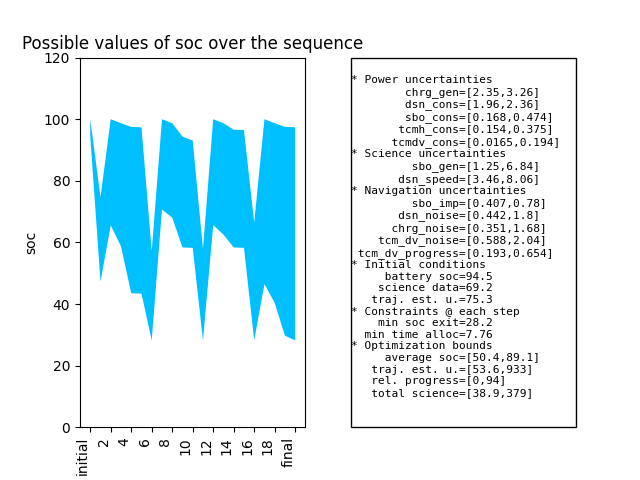Figure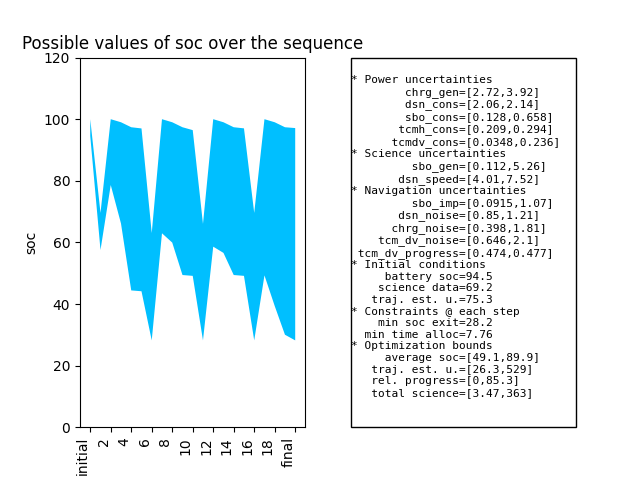Figure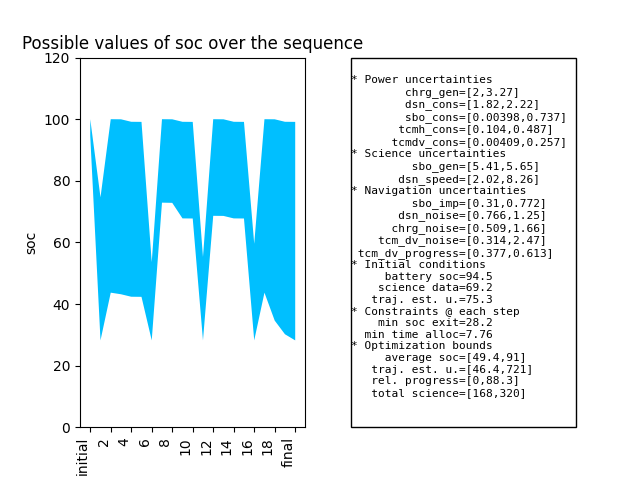Figure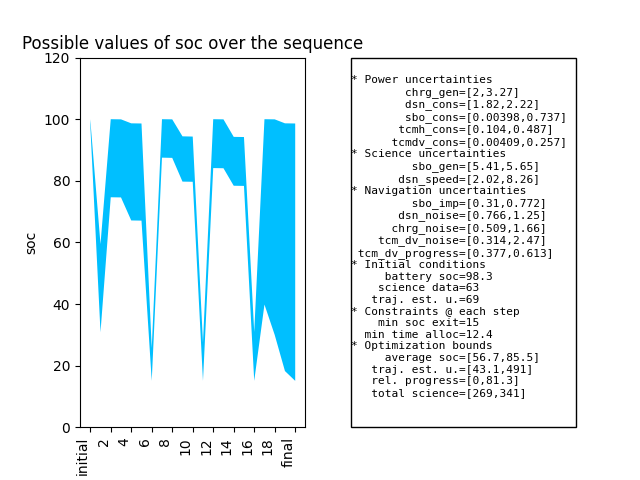Figure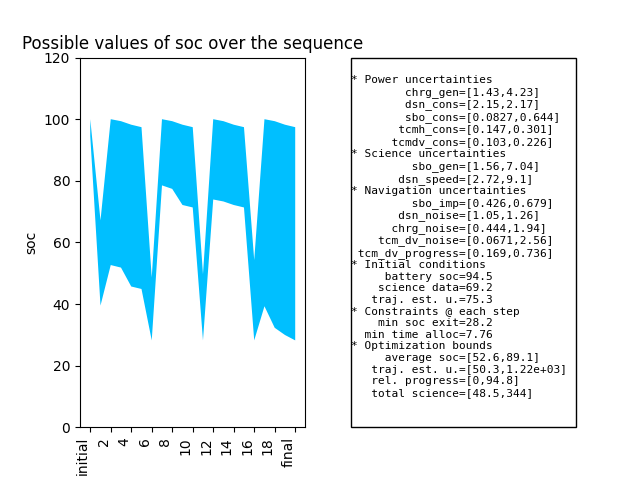Figure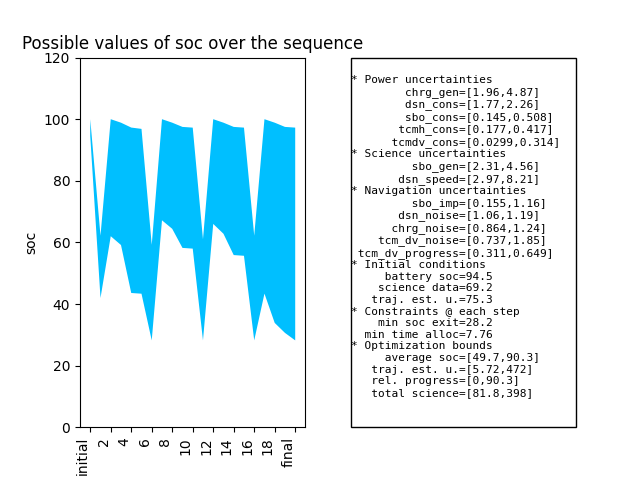Figure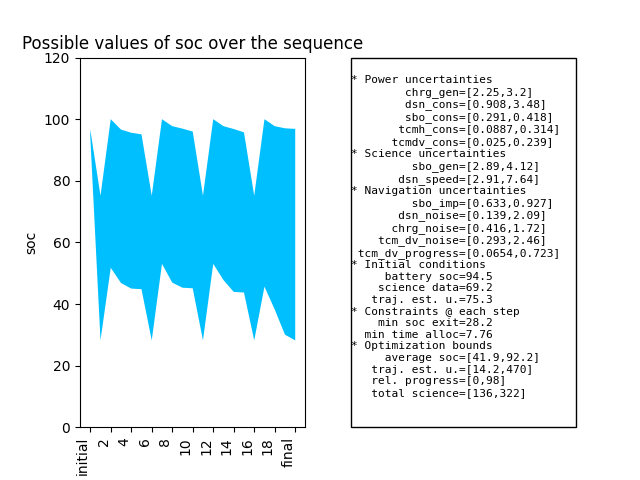Figure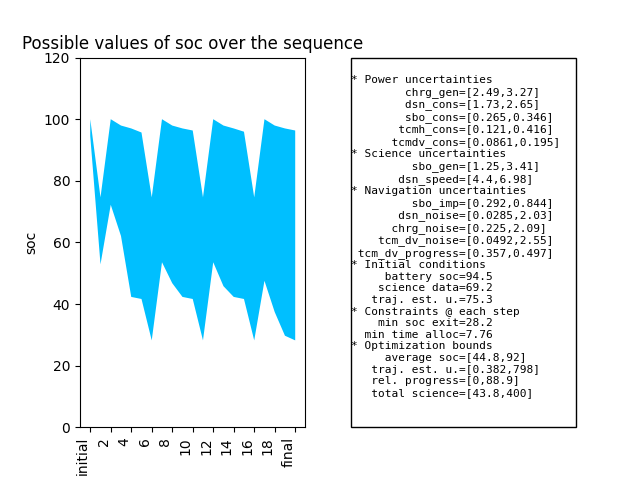Figure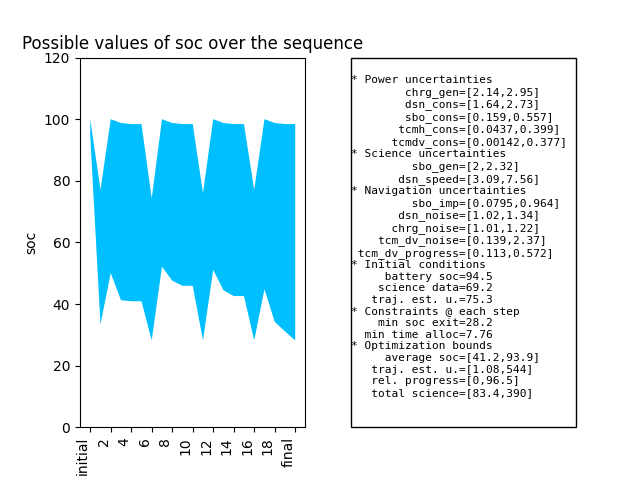Figure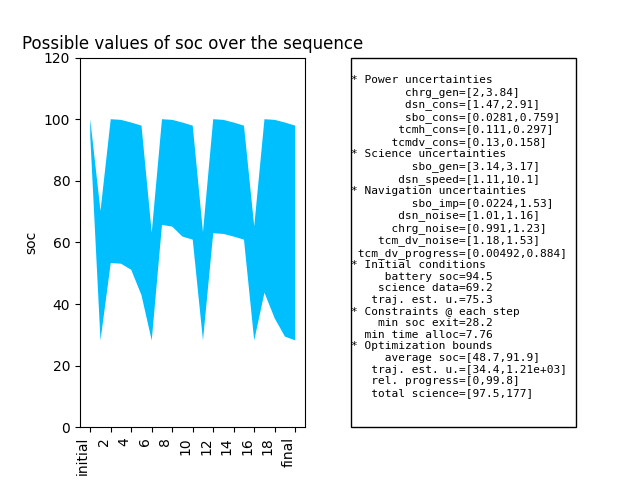Figure